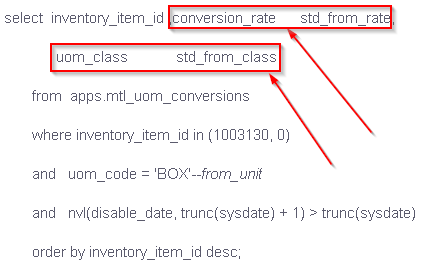Hi Gujjeli,

This could be quite an involved scenario if the UMREZ & UMREN integer values need to be generated in the Oracle SQL query. I'm guessing it would be necessary to write a custom function in Oracle. I'm not familiar with that, but perhaps you can use some logic from some SQL Server functions I once wrote to convert a floating-point number into a numerator and denominator.

`CREATE FUNCTION GetDenominatorFromFloat(@FloatingPoint FLOAT)RETURNS INTASBEGIN--Convert to FractionDECLARE @Denominator INT = POWER(10, LEN(@FloatingPoint) - 1)DECLARE @Numerator INT = @FloatingPoint * @Denominator--Calculate GCFDECLARE @Dividend INT = @DenominatorDECLARE @Divisor INT = @NumeratorDECLARE @Remainder INT = -1WHILE @Remainder != 0BEGINSET @Remainder = @Dividend % @DivisorSET @Dividend = @DivisorSET @Divisor = @RemainderEND--Reduce FractionSET @Denominator /= @Dividend--ReturnRETURN @DenominatorENDGO`
`CREATE FUNCTION GetNumeratorFromFloat(@FloatingPoint FLOAT)RETURNS INTASBEGIN--Convert to FractionDECLARE @Denominator INT = POWER(10, LEN(@FloatingPoint) - 1)DECLARE @Numerator INT = @FloatingPoint * @Denominator--Calculate GCFDECLARE @Dividend INT = @DenominatorDECLARE @Divisor INT = @NumeratorDECLARE @Remainder INT = -1WHILE @Remainder != 0BEGINSET @Remainder = @Dividend % @DivisorSET @Dividend = @DivisorSET @Divisor = @RemainderEND--Reduce FractionSET @Numerator /= @Dividend--ReturnRETURN @NumeratorENDGO`
•From reading your post, it appears that there are pictures. I tried viewing this post on multiple devices, but there are no pictures.

•Sorry images are not being displayed

The code proposed by the client team for extraction

select  inventory_item_id ,conversion_rate      std_from_rate,

uom_class            std_from_class

from  apps.mtl_uom_conversions

where inventory_item_id in (1003130, 0)

and   uom_code = 'BOX'--from_unit

and   nvl(disable_date, trunc(sysdate) + 1) > trunc(sysdate)

order by inventory_item_id desc;

As we can see in the above code they are rounding off the output to the nearest integer to make it compatible to SAP.

But the Problem with the above approach is

• Whenever the function returns the value less than 0.5 then as per the above script the nearest integer would be 0, Which cannot be denominator.
• Please refer 2nd Row from below picture, as we are rounding off 0.5 to 1 as denominator , In case large quantity of Purchases at transactional level the impact will be huge.

Hope this is more clear .

Thanks,

Sailaja

•• Please refer 2nd Row from below picture, as we are rounding off 0.5 to 1 as denominator , In case large quantity of Purchases at transactional level the impact will be huge.

Screenshots have not come through again.

I'm not familiar with Oracle SQL syntax, but are you aliasing these fields?